# C Primer Plus (第五版) 第五章 编程练习 答案

1. 编写一个程序。将用分钟表示的时间转换成以小时和分钟表示的时间。使用#define或者const来创建一个代表60的符号常量。使用while循环来允许用户重复键入值，并且当键入一个小于等于0的时间时终止循环。
#include <stdio.h>
#define M_PER_H	60		// 一小时有60分钟
int main(void)
{
int min,hour,left;		// min:minute, left: 剩余分钟数

printf("请输入分钟数(0或负数终止循环)：\n");
scanf("%d",&min);

while (min > 0)
{
hour = min / M_PER_H
left = min % M_PER_H;

printf("%d minutes are %d hours, %d minutes.\n",min,hour,left);

printf("再一次输入分钟数(0或负数终止循环)：");
scanf("%d",&min);
}

printf("That's all!\n");

return 0;
}
Ouput: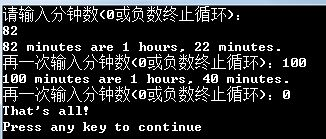2. 编写一个程序，此程序要求输入一整数，然后打印出从（包括）输入的值到（包括）比输入的值大10的所有整数值（也就是说，如果输入为5，那么输出就从5到15）。要求在各个输出值之间用空格、制表符或换行符分。
#include <stdio.h>
int main(void)
{
int num,end;		// end:打印结束的值

printf("请输入一个整数：\n");
scanf("%d",&num);

end = num + 10;

while (num <= end)
{
printf("%d ",num);	// 各输出值之间用空格分开
num++;
}

printf("\n");

return 0;
}

/* 方法2

#include <stdio.h>

int main(void)
{
int num, end;

printf("请输入一个整数：");
scanf("%d", &num);

end = 11;

while (end-- > 0)
{
printf("%d\t", num++);
}

printf("\n");

return 0;
}

*/

/* 方法3

#include <stdio.h>

int main(void)
{
int num, end;
end = 0;

printf("请输入一个整数：");
scanf("%d", &num);

while (end++ < 11)
{
printf("%d\n", num++);
}

printf("\n");

return 0;
}

*/
Output: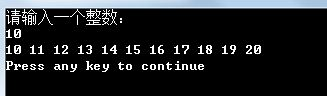3. 编写一个程序，该程序要求用户输入天数，然后将该值转换为周数和天数。例如，此程序将把18天转换成2周4天。用下面的格式显示结果：
18 days are 2 weeks, 4 days.

#include <stdio.h>
#define D_PER_W	7	// 一个星期有7天
int main(void)
{
int days,weeks,left;	// left(left days)

printf("请输入天数(0或负数退出程序)：\n");
scanf("%d",&days);

while (days > 0)
{
weeks = days / D_PER_W;
left = days % D_PER_W;
printf("%d days are %d weeks, %d days.\n",days,weeks,left);
// printf("%d days are %d weeks, %d days.\n",days,days / D_PER_W,days % D_PER_W); 直接输出和先赋值再输出有什么区别

printf("再一次输入天数(0或负数退出程序)：");
scanf("%d",&days);
}

return 0;
}
Output: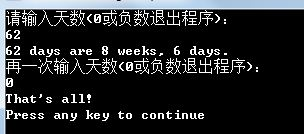4. 编写一个程序让用户按厘米输入一个高度值，然后，程序按照厘米和英尺英寸显示这个高度值。允许厘米和英寸的值出现小数部分。程序允许用户继续输入，直到用户输入一个非正的数值。程序运行的示例如下面所示：
Enter a height in centimeters: 182
182.0 cm = 5 feet, 11.7 inches
Enter a height in centimeters(<=O to quit): 168
168.0 cm = 5 feet, 6.1 inches
Enter a height in centimeters(<=O to quit): 0
bye
#include <stdio.h>
#define C_PER_I 2.54		// 一英寸等于2.54厘米
#define I_PER_F 12		// 一英尺等于12英寸
int main(void)
{
double cm;			// cm = centimeter
double inch;
int feet;

printf("Enter a height in centimeters: ");
scanf("%lf",&cm);

while (cm > 0)
{
inch = cm / C_PER_I;
feet = (int)inch / I_PER_F;

printf("%.1f cm = %d feet, %.1f inches\n",cm,feet,inch);
// printf("%.1f cm = %d feet, %.1f inches\n",cm,feet,inch - feet * I_PER_F); 按照书上思路打印

printf("Enter a height in centimeters (<=0 to quit): ");
scanf("%lf",&cm);
}

printf("bye\n");

return 0;
}
Output: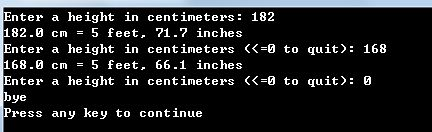5. 改写用来找到前20个整数之和的程序addemup.c（程序清单5.13）（如果您愿意，可以把addemup.c程序看成是一个计算如果您第一天得到$l，第二天得到$2，第三天得到$3，以此类推，您在20天里会挣多少钱的程序）。修改该程序，目的是您能交互地告诉程序计算将进行到哪里。也就是说，用一个读入的变量来代替20。 #include <stdio.h> int main(void) { int count, sum; int num; printf("请输入一个整数，求1到这个数的和:\n"); scanf("%d",&num); count = 0; sum = 0; while (count++ < num) sum = sum + count; printf("sum = %d\n",sum); return 0; } Output: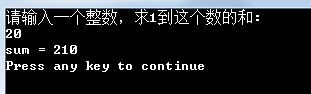6. 现在修改编程练习5中的程序，使它能够计算整数平方的和（如果您喜欢，可以这样认为：如果您第一天得到$l，第二天得到$4，第三天得到$9，以此类推您将得到多少钱。这看起来像一个很好的买卖）。C没有平方函数，但是您可以利用n的平方是n*n的事实。
#include <stdio.h>
int main(void)
{
int count, sum;
int num;

printf("请输入一个整数，求1到这个数的平方和:\n");
scanf("%d",&num);

count = 0;
sum = 0;

while (count++ < num)
sum = sum + count * count;
printf("Square sum = %d\n",sum);

return 0;
}
Output: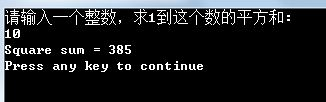7. 编写一个程序，该程序要求输入一个float型数并打印该数的立方值。使用您自己设计的函数来计算该值的立方并且将它的立方打印出来。main()程序把输入的值传递给该函数。
#include <stdio.h>
void cube(float n);
int main(void)
{
float num;

printf("请输入一个浮点数：\n");
scanf("%f",&num);

cube(num);

return 0;
}

void cube(float n)
{
printf("%.2f 的立方是：%.2f\n",n,n * n * n);
}
Output: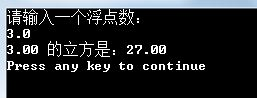8. 编写一个程序，该程序要求用户输入一个华氏温度。程序以double类型读入温度值，并将它作为一个参数传递给用户提供的函数Temperatures()。该函数将计算相应的摄氏温度和绝对温度，并以小数点右边有两位数字的精度显示这三种温度。它应该用每个值所代表的温度刻度来标识这3个值。下面是将华氏温度转换成摄氏温度的方程：
Celsius = 1.8 * Fahreneit + 32.0

Kelvin=Celsius+273.16
Temperatures()函数使用const来创建代表该转换里的3个常量的符号。main()函数将使用一个循环来允许用户重复地输入温度，当用户输入q或其他非数字值时，循环结束。
#include <stdio.h>
void temperatures(double f);
int main(void)
{
double fahrenheit;

printf("请输入一个华氏温度：\n");

while (scanf("%lf",&fahrenheit) == 1)
{
temperatures(fahrenheit);
printf("请输入一个华氏温度(输入q或非数字值，循环结束)：\n");
}

printf("That's all!\n");

return 0;
}

void temperatures(double f)		// 按照书中给出的公式计算，但是公式好像有错，错在将华氏和摄氏弄反
{
const double FTC_S1 = 1.8;		// 华氏温度转换成摄氏温度的转换因子1
const double FTC_S2 = 32.0;		// 华氏温度转换成摄氏温度的转换因子2
const double CTK_S1 = 273.16;	// 摄氏温度转换成绝对温度的转换因子

double celsius,kelvin;

celsius = FTC_S1 * f + FTC_S2;

kelvin = celsius + CTK_S1;

printf("Fahrenheit: %.2f℉\n",f);
printf("Celsius: %.2f℃\n",celsius);
printf("Kelvin: %.2fK\n",kelvin);
}

/* 正确公式的函数

void temperatures(double f)
{
const double FTC_S1 = 1.8;		// 华氏温度转换成摄氏温度的转换因子1
const double FTC_S2 = 32.0;		// 华氏温度转换成摄氏温度的转换因子2
const double CTK_S1 = 273.16;	// 摄氏温度转换成绝对温度的转换因子

double celsius,kelvin;

celsius = (f - FTC_S2) / FTC_S1;

kelvin = celsius + CTK_S1;

printf("Fahrenheit: %.2f℉\n",f);
printf("Celsius: %.2f℃\n",celsius);
printf("Kelvin: %.2fK\n",kelvin);
}

*/
Output: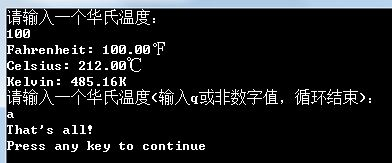12-071109
09-221987
02-28
09-27
11-123935
10-201764
10-19840
12-2122万+
07-301746